# Texas Go Math Grade 4 Lesson 12.5 Answer Key Find the Perimeter and Area

Refer to our Texas Go Math Grade 4 Answer Key Pdf to score good marks in the exams. Test yourself by practicing the problems from Texas Go Math Grade 4 Lesson 12.5 Answer Key Find the Perimeter and Area.

## Texas Go Math Grade 4 Lesson 12.5 Answer Key Find the Perimeter and Area

Essential Question

How can you use the strategy solve a simpler problem to solve perimeter and area problems?
First the given information tells us, figure is Square or Rectangle, for solving area and perimeter problems by applying the formulas as given below:
Rectangle
A = l x w
P = 2(l+w)
Square
A = s x s
P = 4 x s

Unlock the Problem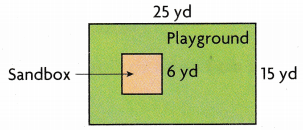A landscaper is laying turf for a rectangular playground. The turf will cover the whole playground except for a square sandbox. How many square yards of turf will the landscaper use?
Answer: 339 square yards of turf will the landscaper use

What do I need to find?
I need to find how many _______________ the landscaper will use.
I need to find how many square yards the landscaper will use.

What information am I given?
The turf will cover the _______________ .
The turf will not cover the _______________.
The length and width of the playground are _______________ and _______________ .
The side length of the square sandbox is _______________ .
The turf will cover the whole playground except for a square sandbox.
The turf will not cover the square box.
The length and width of the playground are 25 yd and 15 yd .
The side length of the square sandbox is 6 yd.

Plan

What is my plan or strategy?
I can solve simpler problems.
Find the area of the _______________ .
Find the area of the _______________ .
Then _______________ the area of the _______________ from the area of the _______________ .
Find the area of the rectangle.
Find the area of the square.
Then subtract the area of the Square from the area of the Rectangle .

Solve

First, find the area of the playground.
A = l × w
= _______________ × _______________
= _______________ square yards
Next, find the area of the sandbox.
A = s × s
= _______________ × _______________
= _______________ square yards
Last, subtract the area of the sandbox from the area of the playground.So, the landscaper will use _______________ of turf to cover the playground.
First, find the area of the playground.
A = l × w
= 25 x 15 = 375 square yards
Next, find the area of the sandbox.
A = s × s
= 6 x 6 = 36 square yards
Last, subtract the area of the sandbox from the area of the playground.So, the landscaper will use 339 Sq yd  of turf to cover the playground.

Try Another ProblemZach is planting a garden for a new museum. A fence will be around the sides of the garden, as shown in the diagram. How many meters of fence does Zach need?

What do I need to find?
How many meters of fence does Zach need?

What information am I given?
length and width of garden. A fence will be around the sides of the garden, as shown in the diagram

Plan

What is my plan or strategy?
Finding the perimeter of the given diagram.

Solve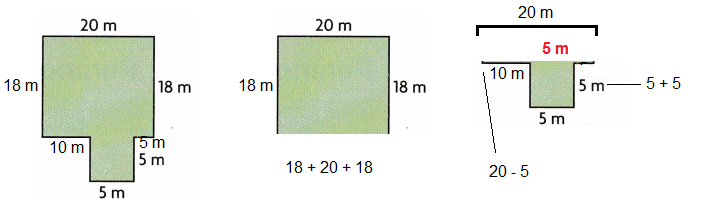Perimeter of the diagram
(18+20+18) + (20-5) +( 5 + 5) = 81 m

How many meters of fence does Zach need? Explain.
81 m
Explanation:to find the perimeter, the sides to be added , pl check above figure
(18+20+18) + (20-5) +( 5 + 5) = 81 m

Share and Show

Question 1.Lila is wall papering one wall of her bedroom, as shown in the diagram. She will cover the whole wall except for the doorway. How many square feet of wallpaper does Lila need?
First, find the area of the wall.
A = l × w = _____________ × _____________ = _____________ square feet
Next, find the area of the door.
A = l × w = _____________ × _____________ = _____________ square feet
Last, subtract the area of the door from the area of the wall.
_____________ – _____________ = _____________ square feet
So, Lila needs _____________ of wallpaper.
First, find the area of the wall.
A = l × w = 12 x 8 = 96 square feet
Next, find the area of the door.
A = l × w = 3 x 7 = 21 square feet
Last, subtract the area of the door from the area of the wall.
96 – 21 = 75 square feet
So, Lila needs 75 of wallpaper.

Question 2.
Ed is building a model of a house with a flat roof, as shown in the diagram. There is a chimney through the roof. Ed will cover the roof with square tiles. If the area of each tile is 1 square inch, how many tiles will he need? Explain.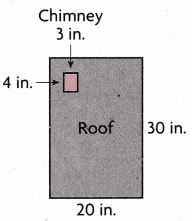588 tiles.
Explanation:
First, find the area of the Roof.
A = l × w = 20 x 30 = 600 square inches
Next, find the area of the Chimney.
A = l × w = 4 x 3 = 12 square inches
Last, subtract the area of the door from the area of the wall.
600 – 12 = 588 square feet
So, Ed needs 588 tiles will he needed

Problem Solving

Question 3.
H.O.T. Multi-Step Mr. Foster is framing two pictures. One is 6 inches by 4 inches and the other is 5 inches by 5 inches. Does he need the same amount of framing for each picture? Explain.
Explanation:
Area of Mr. Foster is framing two pictures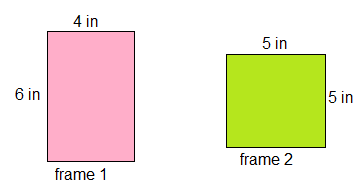frame 1 A =l x w = 6 x 4 =24 square inches
frame 2 A= l x w= 5 x 5 = 25 square inches
one frame is 24 sq inches and another is 25 square inches
one frame is of square as sides are same and another one is of rectangle as sites are different

Question 4.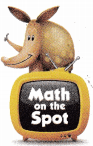H.O.T. Multi-Step Mr. Foster is covering two pictures with glass. One is 6 inches by 4 inches and the other one is 5 inches by 5 inches. Does he need the same number of square inches of glass for each picture? Explain.
Explanation:
frame 1 A =l x w = 6 x 4 =24 square inches of glass
frame 2 A= l x w= 5 x 5 = 25 square inches of glass
one frame is 24 sq inches and another is 25 square inches, one frame is of square as sides are same and another one is of rectangle as sizes are different glass sizes are required

Question 5.
Multi-Step Flynn builds a solar-powered car. The top of the car is shown in the diagram below. He will cover the rectangular top with photocells except for the square that is cut out for the driver’s head. How many square feet will be covered with photocells?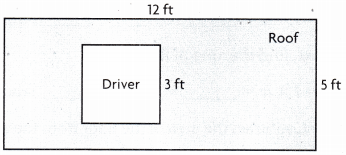(A) 60 square feet
(B) 22 square feet
(C) 11 square feet
(D) 51 square feet
Option (D)
Explanation: A area
A1 = l x w = 12 x 5 = 60 sq ft
A2 = s x s = 3 x 3 = 9 sq ft
Total Area (A1 – A2) = 60 – 9 = 51 square feet

Question 6.
Use a Diagram Julie is putting a fence around part of her yard for a dog run. The part she wants to fence is 25 meters long and 16 meters wide. How many meters of fencing will Julie need?
(A) 400 meters
(B) 82 meters
(C) 380 meters
(D) 64 meters
Option(B)
Explanation:
length = 25; width = 16
Perimeter P= 2(l + w)
p = 2(25 + 16) = 82 m

Question 7.
Multi-Step Use a Diagram Connor is covering his living room floor with square tiles that are each 1 square foot. There is a fireplace on one side of the room that won’t be tiled. How many tiles does Connor need for the floor?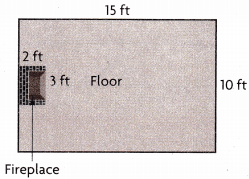(A) 144 tiles
(B) 20 tiles
(C) 44 tiles
(D) 35 tiles
Option(A)
Explanation:
A1 = l x w = 15 x 10 = 150 sq ft
A2 = l x w = 2 x 3 = 6 sq ft
Total Area (A1 – A2) = 150 – 6 = 144 sq feet

TEXAS Test Prep

Question 8.
A rectangular floor is 12 feet long and 11 feet wide. A rug that is 9 feet long and 7 feet wide covers part of the floor. How many square feet of the floor are NOT covered by the rug?
(A) 63 square feet
(B) 69 square feet
(C) 132 square feet
(D) 195 square feet
Option (B)
Explanation:
A1= l x w =12 x 11 = 132 sq ft
A2 = l x w = 9 x 7 = 63 sq ft
Total Area (A1 – A2) = 132 – 63 = 69 sq ft

### Texas Go Math Grade 4 Lesson 12.5 Homework and Practice Answer Key

Problem Solving

Question 1.
Volunteers are fencing a part of City Park for toddlers. The diagram shows the area of a park that will be fenced. How many feet of fencing will be used?Explanation:
Perimeter P = addition of sides lengths of the given figure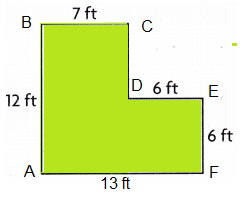P = AB + BC + CD+ EF+ FA
= 12 + 7 + 6 + 6 + 13
= 44 ft

Question 2.
Sam is carpeting a room. The size of the room is shown in the diagram. How many square feet of carpet does Sam need?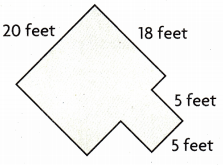385 sq ft square feet of carpet Sam need
Explanation:Total carpet area = A1 + A2
Rectangle ABCD area A1 = l x w = 20 x 18 = 360 sq ft
Square PQRS area A2 = s x s = 5 x 5 = 25 sq ft
square feet of carpet does Sam needed is A = A1+ A2
A = 360 + 25 = 385 sq ft

Question 3.
Grace is helping her teacher cover the classroom door with paper, as shown in the diagram. She will cover the whole door except for the window. How many square feet of paper does Grace need?17 Sq ft
Explanation: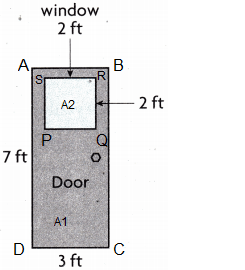Rectangle ABCD area A1 = l x w = 7 x 3 = 21 sq ft
Square area PQRS A2 = s x s = 2 x 2 = 4 sq ft
square feet of carpet does Sam needed is A = A1 – A2
A = 21 – 4 = 17 sq ft

Lesson Check

Question 4.
Mr. Floyd is laying grass squares in his back yard. He will cover the entire yard except for the vegetable garden.How many square feet of grass will be used?
(A) 439 square feet
(B) 112 square feet
(C) 375 square feet
(D) 311 square feet
Option(D)
Explanation:
Rectangle ABCD area A1 = l x w = 25 x 15 = 375 sq ft
Square area PQRS A2 = s x s = 8 x 8 = 64 sq ft
square feet of carpet does Sam needed is A = A1 – A2
A = 375 – 64 = 311 sq ft

Question 5.
Builders are covering a wall that is 4 meters tall and 7 meters wide with bricks. They will cover the entire wall except for a square window that measures 2 meters on all sides.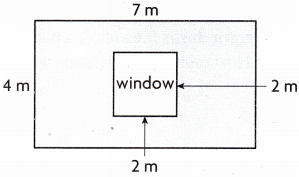How many square meters of the wall will be covered in bricks?
(A) 28 square meters
(B) 32 square meters
(C) 24 square meters
(D) 25 square meters
Option(C)
Explanation:Explanation: 24 sq ft
Rectangle ABCD area A1 = l x w = 7 x 4 = 28 sq ft
Square area PQRS A2 = s x s = 2 x 2 = 4 sq ft
square feet of carpet does Sam needed is A = A1 – A2
A = 28 – 4 = 24 sq ft

Question 6.
Multi-Step A rectangular field is 120 yards long and 53 yards wide. A tent that is 20 yards long and 18 yards wide covers part of the field. How many square yards of the field are NOT covered by the tent?
(A) 6,000 square yards
(B) 6,360 square yards
(C) 422 square yards
(D) 706 square yards
Option (A)
Explanation:
Rectangle area A1 = l x w = 120 x 53 = 6360 sq yds
Square area A2 = l x w = 20 x 18 = 360 sq yds
square feet of carpet does Sam needed is A = A1 – A2
A = 6360 – 360 = 6000 sq yds

Question 7.
Multi-Step Jason is adding a baseboard trim around the edge of his floor. The room is 14 feet wide and 20 feet long. There is a door opening that is 3 feet wide. How many feet of trim will Jason need?
(A) 68 feet
(B) 65 feet
(C) 277 feet
(D) 280 feet